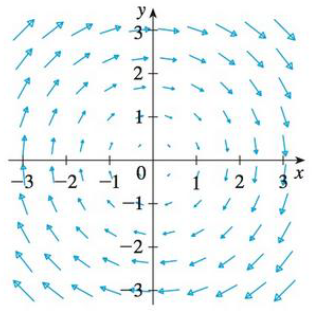Chapter 16.2, Problem 17E

Chapter
Section
Textbook Problem

Let F be the vector field shown in the figure.(a) If C1 is the vertical line segment from (−3, −3) to (−3, 3), determine whether ∫ c 1 F ⋅ d r is positive, negative, or zero.(b) If C2 is the counterclockwise-oriented circle with radius 3 and center the origin, determine whether ∫ c 2 F ⋅ d r is positive, negative, or zero.(a)

To determine

Whether the expression C1Fdr is positive, negative, or zero.

Explanation

The expression C1Fdr is positive.

Given data:

The curve C1 is the line segment from the point (3,3) to (3,3) .

Formula used:

Write the expression CFdr for a curve C .

CFdr=CFTds (1)

Here,

T is the unit tangent vector.

Refer to Figure in the textbook (i.e., bottom of the question 16.2-17E)

(b)

To determine

Whether the expression C2Fdr is positive, negative, or zero.

Still sussing out bartleby?

Check out a sample textbook solution.

See a sample solution

The Solution to Your Study Problems

Bartleby provides explanations to thousands of textbook problems written by our experts, many with advanced degrees!

Get Started